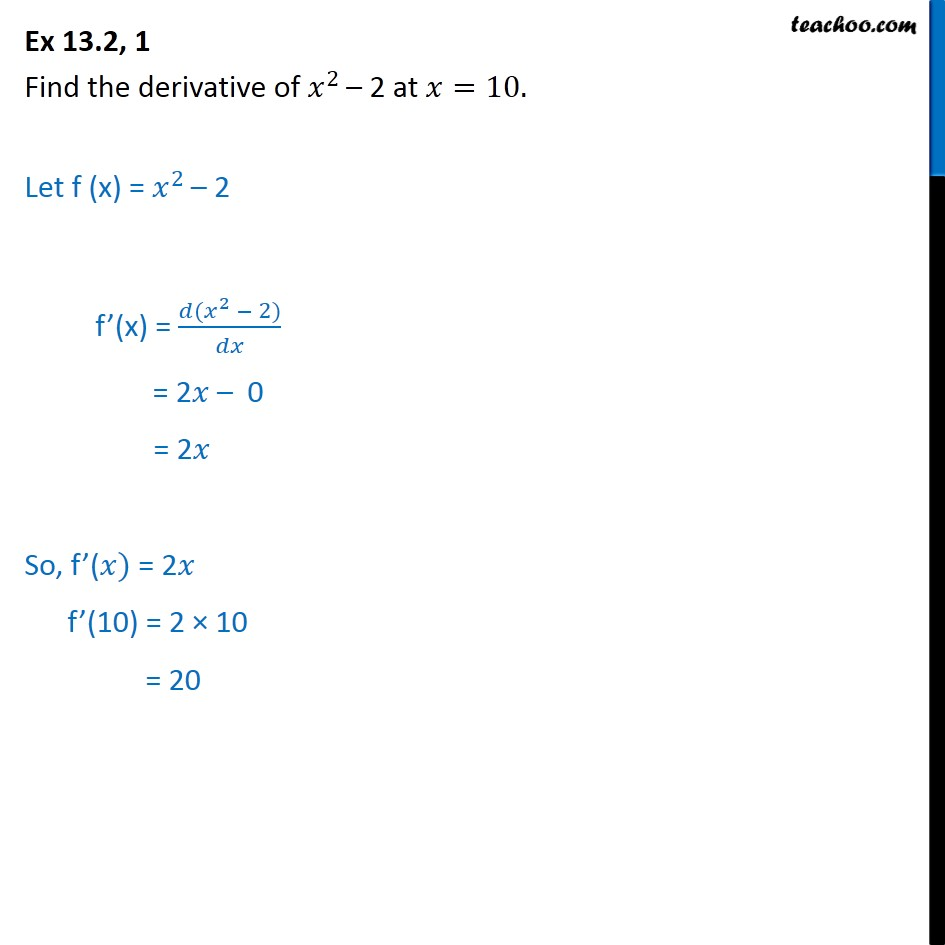1. Chapter 13 Class 11 Limits and Derivatives (Term 1 and Term 2)
2. Serial order wise
3. Ex 13.2 (Term 2)

Transcript

Ex 13.2, 1 Find the derivative of 𝑥﷮2 ﷯– 2 at 𝑥=10. Let f (x) = 𝑥﷮2 ﷯– 2 f’(x) = 𝑑( 𝑥﷮2﷯ − 2)﷮𝑑𝑥﷯ = 2𝑥 – 0 = 2𝑥 So, f’(𝑥) = 2𝑥 f’(10) = 2 × 10 = 20

Ex 13.2 (Term 2)